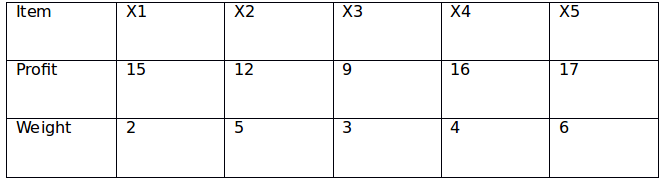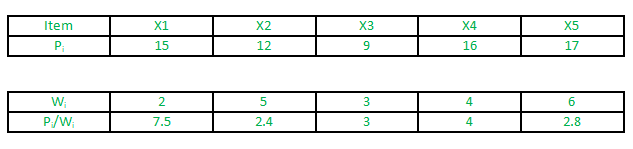# GATE | GATE 2017 MOCK II | Question 26

• Last Updated : 14 Feb, 2018

Consider the following instance of knapsack problem:The maximum weight of 12 is allowed in the knapsack.

Find the value of maximum profit with the optimal solution of the fractional knapsack problem.
(A) 31
(B) 40.2
(C) 48.5
(D) None of these

Explanation:Decreasing order of P i /W i is

X1, X4, X3, X5, X2

X1 –> profit = 15 and weight = 2

Including X4 –> profit = 15 + 16 and weight = 2 + 4 = 6

Including X3 –> profit = 40 and weight = 9

Now weight left = 3

Weight of X5 = 6 –> half of X5 can be included.

Profit = 40 + 17/2 = 48.5.

Quiz of this Question

My Personal Notes arrow_drop_up
Recommended Articles
Page :